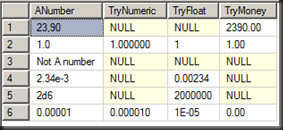# Goodbye IsNumeric hell

A well overdue feature introduced in Denali CTP 3 is that of the Try_Parse and Try_Convert functions. These are great for dealing with the something that’s frustrated SQL developers for years – data type conversions.

Let’s imagine a rather nasty case, a file with some values in it that once imported into SQL (as character data) looks something like this:Ewww… that’s a mess. Let’s see if we can identify which of the values can be converted into a numeric data type. The function prior to Denali for that was ISNUMERIC.

```SELECT ANumber, ISNUMERIC(ANumber)Great, so other than the obvious one, they’re all numeric data. Time to get converting.

```SELECT CAST(ANumber as Numeric(18,6))
WHERE ISNUMERIC(ANumber) = 1;```

Msg 8114, Level 16, State 5, Line 1
Error converting data type varchar to numeric.

Err, so they’re numeric but can’t be converted to numeric. That’s fun. Maybe another of the numeric data types will work…

```SELECT CAST(ANumber as FLOAT)
WHERE ISNUMERIC(ANumber) = 1;```

Msg 8114, Level 16, State 5, Line 1
Error converting data type varchar to float.

Not that one either. Money perhaps, it’s usually more forgiving than float or numeric.

```SELECT CAST(ANumber as MONEY)
WHERE ISNUMERIC(ANumber) = 1;```

Msg 235, Level 16, State 0, Line 1
Cannot convert a char value to money. The char value has incorrect syntax.

Different error, same problem. The issue here is that there’s no single data type that all of those messy values can convert to. 23,90 can convert to money, not float or numeric. 2.34e-3 and 2d6 both convert fine to float, not to money or numeric.

So ISNUMERIC is a bit of a misleading function. All it tells you is that the value can be cast to one of the numeric data types in SQL, it’s up to you to figure out which one.

Pre-Denali a data set like that would be a terrible mess to get converted (though I’d probably just send it back to where it came from and ask for it to be cleaned at the source). In Denali though, it’s not all that bad.

```SELECT ANumber, TRY_CONVERT(NUMERIC(18,6), ANumber)Now that’s more like it… We can do the same with the other numeric data types

```SELECT ANumber,
TRY_CONVERT(NUMERIC(18,6), ANumber) as TryNumeric,
TRY_CONVERT(FLOAT, ANumber) AS TryFloat,
TRY_CONVERT(MONEY, ANumber) AS TryMoneyOther than the one that’s clearly not a number of any form, the rest convert to at least one of the data types, and now I have an error-free way to convert everything that converts and ignore the rest without having to write nasty validation functions of my own.

```WITH ConvertAMess (Original, ConvertedNumber) AS (
SELECT ANumber,
COALESCE(TRY_CONVERT(NUMERIC(18,6), ANumber),TRY_CONVERT(FLOAT, ANumber),TRY_CONVERT(MONEY, ANumber))
)
SELECT  Original, ConvertedNumber
FROM ConvertAMess
WHERE ConvertedNumber IS NOT NULL;```Not perfect, but a darn side better than what we started with. That’s going to simplify the kind of data-cleansing imports that I’ve been seeing recently.

1.Gordon

for this I used a clr function that basically does the same as Try_Convert functions

2.You missed the two most egregious ones. isnumeric(‘-‘) and isnumeric(‘.’) both also return true (1). isnumeric() is the most useless function there is in SQL Server.

Same as Gordon, I implemented these years ago in CLR functions as well as a real version of IsNumeric() that only returns true if there’s a valid numeric conversion.

Interesting that you left out a conversion to DECIMAL? All of your numeric examples are valid decimals.

3.Gail (Post author)

Yeah, I left those out. I don’t even want to think of them…

Numeric and decimal are the same data type. One’s just an alias of the other. In fact, if you try to cast to decimal and it fails, the error says ‘error converting to numeric’

SELECT CAST(‘2.34e02’ as DECIMAL(18,6))
returns
Msg 8114, Level 16, State 5, Line 1
Error converting data type varchar to numeric.

4.Joe Celko

Great article! But I am bothered by the idea that stinking bad data can get into the Datasbase. Maybe we need an ANSI/ISO DSL (Data Scrubbing Language)as an ETL in the Standard toadd to the DDL, DML and DCL?

5.Gail (Post author)

It’s not necessarily in the database. My example was a table, but it could have been an openquery to a spreadsheet in real life.

Normally doing data import I’ll import data raw into a database table (a staging environment) via something like a bcp in, and clean it once in SQL. It’s often easier than trying to clean via whatever import tool is being used.

6.Frank Hell

Great! ISNUMERIC was always pain in the a**.

7.Simran Jindal

Great Article! And I totally agree with your comment on importing the data into a staging environment and then doing the data cleaning. It’s just not worth the trouble to do import and data cleaning at the same time.

This site uses Akismet to reduce spam. Learn how your comment data is processed.